The cohomological dimensionof a topological spacerelative to the group of coefficientsis the maximum integerfor which there exists closed subsetsofsuch that the cohomology groups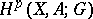are non-zero. The homological dimensionis similarly defined (cf. Homological dimension of a space). Finite Lebesgue dimension (covering dimension) is the same as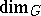(or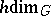) ifis the subgroup of the integers (or real numbers modulo 1). In Euclidean space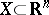the equation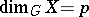is equivalent to the property thatis locally linked by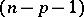-dimensional cycles (with coefficients in). For paracompact spaces, the inequality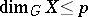is equivalent to the existence of soft resolutions (cf. Soft sheaf and Resolution) forof length. Since soft sheaves are acyclic, in this way a connection is established with the general definition of dimension in homological algebra; for example, the injective (or projective) dimension of a module isif it has an injective (or projective) resolution of length; the global dimension of a ring is the maximum of the injective (or projective) dimensions of the modules over the ring and is the analogue of the Lebesgue dimension of.